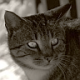## Pretty Printing a Tree in a Terminal

More often than I’d like, simple tasks turn out to be not that simple. For example, displaying (beautifully) a binary tree for debugging purpose in a terminal. Of course, one could still use lots of parentheses, but that does not lend itself to a very fast assessment of the tree. We could, however, use box drawing characters, as in DOS’s goode olde tymes, since they’re now part of the Unicode standard.A first try might look something like this:

```void p_show(node<K,T> * r) const
{
if (r)
{
std::cout
<< "([" << r->key << "," << r->data << "]";

p_show(r->left,b);
p_show(r->right,b);
std::cout << ")";
}
else
std::cout << "()";
}
```

This simple recursive procedure vomits:

```([triceratops,3]([ornithorynque,5]()([pleiosaure,12]()([rhinoceros,0]()())))([zebre,-4]([urubu,15]()())([zygoptera,2]([zybyg,0]()())())))
```

That’s really hard to read fast. We must squint and count parentheses to see what’s what where. We can do better, at least something to show the actual structure of the tree. We could, for each item use a different line and indent by depth. That’s not complicated: we pass an additional depth argument to the function, call it with +1 for the next level, and use something like std::setw(depth) to print the appropriate number of space before the lines.

```void p_show_3(node<K,T> * r,int depth) const
{
if (r)
{
std::cout
<< std::setw(depth) << " "
<< "([" << r->key << "," << r->data << "]"
<< std::endl;

p_show_3(r->left,depth+1);
p_show_3(r->right,depth+1);
}
else
std::cout
<< std::setw(depth+1) << "X"
<< std::endl;
}
```

This produces the already much better:

``` [triceratops,3]
[ornithorynque,5]
X
[pleiosaure,12]
X
[rhinoceros,0]
X
X
[zebre,-4]
[urubu,15]
X
X
[zygoptera,2]
[zybyg,0]
X
X
X
```

The Xs mark nullptrs, just as in the previous function () did. We now have the general structure of the tree, but we can make it still a bit cuter. Let’s add lines connecting explicitly items. That’ll be especially useful when the tree grows and a node’s sibling is several lines away. To so so without a GUI, we must resort to the retro-awesome goodness of box-drawing characters. Fortunately, every kind-of-modern terminal with a Unicode-capable font will do.

```using bits=std::vector<bool>;

void p_tabs(const bits & b) const
{
for (auto x: b)
std::cout << (x?" \u2502":"  ");
}

void p_show(node<K,T> * r, bits & b) const
{
// https://en.wikipedia.org/wiki/Box-drawing_character
if (r)
{
std::cout
<< "[" << r->key << "," << r->data << "]"
<< std::endl;

p_tabs(b);
std::cout << " \u251c"; // ├
b.push_back(true);
p_show(r->left,b);
b.pop_back();

p_tabs(b);
std::cout << " \u2514"; // └
b.push_back(false);
p_show(r->right,b);
b.pop_back();
}
else
std::cout << " \u25cb" << std::endl; // ○
}

void show() const { bits b; p_show(root,b); }
```

This produces:

```[triceratops,3]
├[ornithorynque,5]
│ ├ ○
│ └[pleiosaure,12]
│   ├ ○
│   └[rhinoceros,0]
│     ├ ○
│     └ ○
└[zebre,-4]
├[urubu,15]
│ ├ ○
│ └ ○
└[zygoptera,2]
├[zybyg,0]
│ ├ ○
│ └ ○
└ ○
```

How does the algorithm work? Why do we need a bit vector? The bit vector is a simple way of keeping track of the vertical lines. At depth x, we must draw a vertical line from the left to the right sibling, regardless of how many nodes there are in the left sibling’s sub-tree. At depth x, we push a 1 bit on the bit vector/stack, and that bit remains 1 until we reach the right sibling, also at depth x, which sets the bit to zero. The vertical lines of the right sub-tree will all be at depth x+1 and beyond, so they are handled recursively. Lovely and simple, isn’t it.

*
* *

The problem of pretty-printing a tree in graphic mode where the tree should look good is a lot more complicated, even when the tree isn’t too big. It’s difficult because we can’t really define what “pretty” means in this particular case. Is it when the tree is nicely spaced using powers of two? Is it when a sub-tree can use an space left in another sub-tree to have it more compact? A bit a both?

### 3 Responses to Pretty Printing a Tree in a Terminal

1.Jake S says:

This is an awesome simple way to print trees, thank you for the contribution! I’ve looked at the final program multiple times and I think it prints trees mirrored or reversed. I tried it with just a simple 3 node tree and the left is on the right and visa versa. It’s a pretty simple fix though.

2.ibrahim chegrane says:

Thank you for the code, it works very well.
I made a small change for N-ary tree, and it works well.

```
using bits=std::vector;

void p_tabs(const bits & b)
{
for (auto x: b)
//std::cout << (x?" \u2502":"  ");
std::cout << (x?" |":"  ");
}

//void p_show(node * r, bits & b) const
void p_show(Node *r, bits &b)
{
// https://en.wikipedia.org/wiki/Box-drawing_character
if (r)
{
std::cout
<< "[" <cara << "," <listChildern << "]"
<< std::endl;

for (int i = 0; i <NB_CHAR-1 ; ++i)
{
p_tabs(b);
///std::cout << " \u251c"; // ├
std::cout <listChildern[i],b);
b.pop_back();
}

p_tabs(b);
//std::cout << " \u2514"; // └
std::cout <listChildern[NB_CHAR-1],b);
b.pop_back();
}
else
//std::cout << " \u25cb" << std::endl; // ○
std::cout << " o" << std::endl; // ○
}

void show(Node *root)
{
bits b;
p_show(root,b);
}

```

To solve the problem of the Unicode character, you can use this web page to find all the code according to the encoding and the language used.

https://charbase.com/251c-unicode-box-drawings-light-vertical-and-right

ibrahim chegrane’

•Steven Pigeon says:

Thanks!